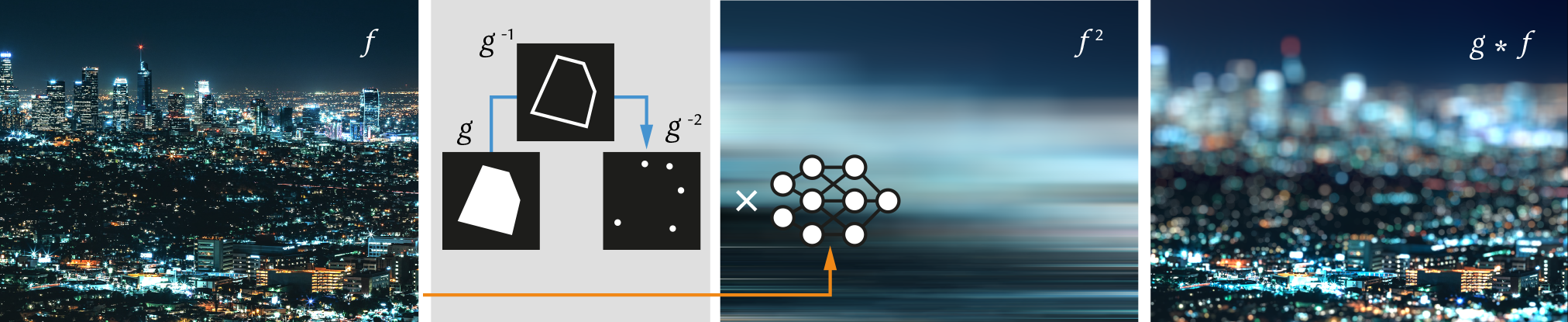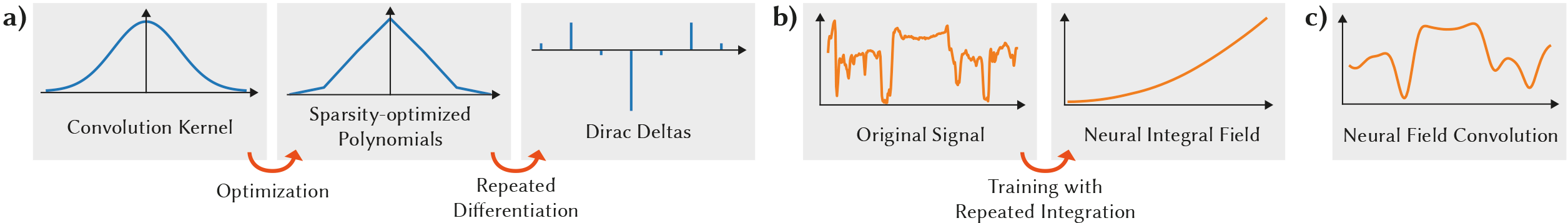# Neural Field Convolutions by Repeated Differentiation

## ACM Transactions on Graphics (Proceedings of SIGGRAPH Asia), 2023

1Max-Planck-Institut für Informatik, 2University College LondonWe introduce an algorithm to perform efficient continuous convolution of neural fields 𝑓 by piecewise polynomial kernels 𝑔. The key idea is to convolve the sparse repeated derivative of the kernel with the repeated antiderivative of the signal.

## Abstract

Neural fields are evolving towards a general-purpose continuous representation for visual computing. Yet, despite their numerous appealing properties, they are hardly amenable to signal processing. As a remedy, we present a method to perform general continuous convolutions with general continuous signals such as neural fields. Observing that piecewise polynomial kernels reduce to a sparse set of Dirac deltas after repeated differentiation, we leverage convolution identities and train a repeated integral field to efficiently execute large-scale convolutions. We demonstrate our approach on a variety of data modalities and spatially-varying kernels.

## Methoda) Given an arbitrary convolution kernel, we optimize for its piecewise polynomial approximation, which under repeated differentiation yields a sparse set of Dirac deltas. b) Given an original signal, we train a neural field which captures the repeated integral of the signal. c) The continuous convolution of the original signal and the convolution kernel is obtained by a discrete convolution of the sparse Dirac deltas from a) and corresponding sparse samples of the neural integral field from b).

## Animation

### We consider the task of filtering a Neural Field representation of a noisy motion-capture sequence which consists of 23 3D joint position paths over time resulting in a field mapping time to joint position. We obtain smooth motion trajectories by applying a Gaussian Filter to the Neural representation.

Left: Original noisy motion capture data. Right: Our filtered result.

## Audio

### Audio Signal can be represented using Neural Fields mapping time to sound intensity. Below we apply our framework to an audio signal.

Left: Original. Center: Low-pass reference. Right: Our low-pass filtered result.

## BibTeX

``````
@article{Nsampi2023NeuralFC,
author = {Ntumba Elie Nsampi and Adarsh Djeacoumar and Hans-Peter Seidel and Tobias Ritschel and Thomas Leimk{\"u}hler},
title = {Neural Field Convolutions by Repeated Differentiation},
year = {2023},
issue_date = {December 2023},
publisher = {Association for Computing Machinery},